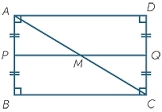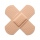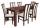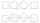# The circumference

The circumference and width of the rectangle are in a ratio of 5: 1. its area is 216cm2. What is its length?

a =  18 cm

### Step-by-step explanation:Did you find an error or inaccuracy? Feel free to write us. Thank you!Tips to related online calculators
Check out our ratio calculator.
Do you have a linear equation or system of equations and looking for its solution? Or do you have a quadratic equation?

#### You need to know the following knowledge to solve this word math problem:

We encourage you to watch this tutorial video on this math problem:

## Related math problems and questions:

• The rectangleDetermine the area of the rectangle where the length and width are in the ratio 5:2 and its length is 7.5 cm longer than its width. Determine also its length and its width.
• RectangleThe length of the rectangle are in the ratio 5:12 and the circumference is 238 cm. Calculate the length of the diagonal and area of rectangle.
• The sidesThe sides of the rectangle are in a ratio of 3: 5 and its circumference measures 72 cm. Calculate: a) the size of both sides of the rectangle b) the area of the rectangle c) the length of the diagonals
• RectangleThe length of the rectangle is 12 cm greater than 3 times its width. What dimensions and area this rectangle has if ts circumference is 104 cm.
• RectanglePerimeter of rectangle is 48 cm. Calculate its dimensions if they are in the ratio 5:3 (width:height)
• Two patchesPeter taped the wound with two rectangular patches (one over the other to form the letter X). The area sealed with both patches at the same time had a content of 40cm2 and a circumference of 30cm. One of the patches was 8cm wide. What was the width of the
• TableThe circumference of the rectangle table is 420 cm. Length to width ratio is 5:2 . Calculate table dimensions and dimensions reduced in the ratio 3:5
• Rectangle diagonalsIt is given a rectangle with an area of 24 cm2 a circumference of 20 cm. The length of one side is 2 cm larger than the length of the second side. Calculate the length of the diagonal. Length and width are yet expressed in natural numbers.
• RectangleThe width of the rectangle is 65% of its length. Perimeter of the rectangle is 132 cm. Determine the dimensions of the rectangle.
• Ratio of sidesCalculate the area of a circle with the same circumference as the circumference of the rectangle inscribed with a circle with a radius of r 9 cm so that its sides are in ratio 2 to 7.
• The sidesThe sides of a rectangle are in a ratio of 2:3, and its perimeter is 1 1/4 inches. What are the lengths of its side? Draw it.
• In theIn the rectangle ABCD, the distance of its center from the line AB is 3 cm greater than from the line BC. The circumference of the rectangle is 52 cm. Calculate the contents of the rectangle. Express the result in cm2.
• Perimeter of a rectangleIf the perimeter of a rectangle is 114 meters and the length is twice the width plus 6 meters, what are the length and width?
• Square to rectangleWhat is the ratio of the area of a square of side x to the area of a rectangle of a rectangle of width 2 x and length 3
• Dimensions of rectangleFind the dimensions of a rectangle given that its perimeter is 110 cm and its length is 1 cm more than twice its width.
• The sides 2The sides of a trapezoid are in the ratio 2:5:8:5. The trapezoid's area is 245. Find the height and the perimeter of the trapezoid.
• Ratio of sidesThe triangle has a circumference of 21 cm, and the length of its sides is in a ratio of 6: 5: 3. Find the length of the longest side of the triangle in cm.